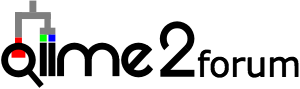# qiime2R: changing facets

Hi, thank you for your very useful package.

I am in the middle of making my heatmap, I change several script to suit my data.
Now I am wondering the possibility of changing the order of the facet. Could you change the order of the body_site of the facet?
For example, in your attached figure, they were ordered like gut;left palm;right palm;tongue. What should be added to the script/command to change it in custom order? E.g right palm;left palm; tongue;gut ??

I try the factor() function with levels the “body_site” but error that dollar sign are not accepted in atomic vector appeared.

Thank you.

You have the right idea, the line you would want is:

``````mutate(`body-site`=factor(`body-site`, levels=c("right palm","left palm","gut","tongue")))
``````

Perhaps it was an issue relating to the “-”?

Full code below:

``````
library(tidyverse)
library(qiime2R)

SVs<-apply(SVs, 2, function(x) x/sum(x)*100) #convert to percent

SVsToPlot<-
data.frame(MeanAbundance=rowMeans(SVs)) %>% #find the average abundance of a SV
rownames_to_column("Feature.ID") %>%
arrange(desc(MeanAbundance)) %>%
top_n(30, MeanAbundance) %>%
pull(Feature.ID) #extract only the names from the table

SVs %>%
as.data.frame() %>%
rownames_to_column("Feature.ID") %>%
gather(-Feature.ID, key="SampleID", value="Abundance") %>%
mutate(Feature.ID=if_else(Feature.ID %in% SVsToPlot,  Feature.ID, "Remainder")) %>% #flag features to be collapsed
group_by(SampleID, Feature.ID) %>%
summarize(Abundance=sum(Abundance)) %>%
mutate(NormAbundance=log10(Abundance+0.01)) %>% # do a log10 transformation after adding a 0.01% pseudocount. Could also add 1 read before transformation to percent
left_join(taxonomy) %>%
mutate(Feature=paste(Feature.ID, Taxon)) %>%
mutate(Feature=gsub("[kpcofgs]__", "", Feature)) %>% # trim out leading text from taxonomy string
mutate(`body-site`=factor(`body-site`, levels=c("right palm","left palm","gut","tongue"))) %>%
ggplot(aes(x=SampleID, y=Feature, fill=NormAbundance)) +
geom_tile() +
facet_grid(~`body-site`, scales="free_x") +
theme_q2r() +
theme(axis.text.x=element_text(angle=45, hjust=1)) +
scale_fill_viridis_c(name="log10(% Abundance)")
ggsave("heatmap.pdf", height=4, width=11, device="pdf") # save a PDF 3 inches by 4 inches
``````

Thank you! It works!
I initially try it like this

mutate(SVs\$body-site=factor(SVs\$body-site, levels=c(“right palm”,“left palm”,“gut”,“tongue”)))
something like that, and I dont know about where to put the code. Thanks

That would have worked perfectly if you were not using the pipe/mutate function!